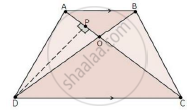Share

Books Shortlist
Your shortlist is empty

# Solution for Abcd is a Trapezium in Which Ab || Cd. the Diagonals Ac and Bd Intersect at O. Prove That: (I) Triangle Aob and Triangle Cod (Ii) If Oa = 6 Cm, Oc = 8 Cm, Find:(A) ("Area"(Triangleaob))/("Area"(Trianglecod)) (B) ("Area"(Triangleaod))/("Area"(Trianglecod)) - CBSE Class 10 - Mathematics

#### Question

ABCD is a trapezium in which AB || CD. The diagonals AC and BD intersect at O. Prove that: (i) ΔAOB and ΔCOD (ii) If OA = 6 cm, OC = 8 cm,

Find:(a)  ("area"(triangleAOB))/("area"(triangleCOD))

(b)  ("area"(triangleAOD))/("area"(triangleCOD))

#### SolutionWe have,

AB || DC

In ΔAOB and ΔCOD

∠AOB = ∠COD [Vertically opposite angles]

∠OAB = ∠OCD [Alternate interior angles]

Then, ΔAOB ~ ΔCOD [By AA similarity]

(a) By area of similar triangle theorem

("area"(triangleAOB))/("area"(triangleCOD))="OA"^2/"OC"^2=6^2/8^2=36/64=9/16

(b) Draw DP ⊥ AC

therefore("area"(triangleAOD))/("area"(triangleCOD))=(1/2xxAOxxDP)/(1/2xxCOxxDP)

="AO"/"CO"=6/8=3/4

Is there an error in this question or solution?

#### Video TutorialsVIEW ALL 

Solution for question: Abcd is a Trapezium in Which Ab || Cd. the Diagonals Ac and Bd Intersect at O. Prove That: (I) Triangle Aob and Triangle Cod (Ii) If Oa = 6 Cm, Oc = 8 Cm, Find:(A) ("Area"(Triangleaob))/("Area"(Trianglecod)) (B) ("Area"(Triangleaod))/("Area"(Trianglecod)) concept: Areas of Similar Triangles. For the course CBSE
S